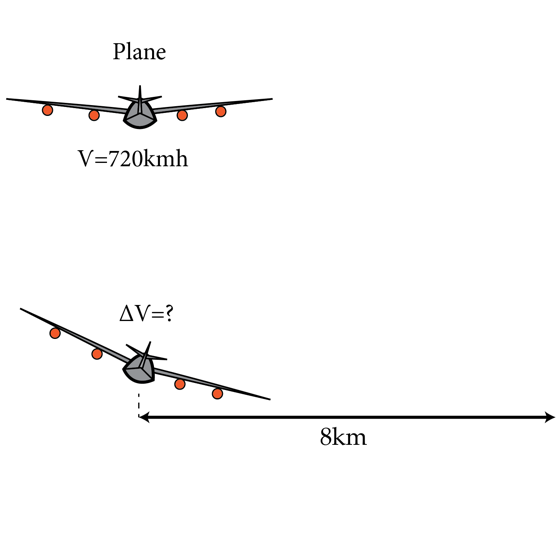A simple flying maneuver

A plane flies horizontally at constant ground speed $v=720 \textrm{km/h}$. The pilot is informed by an air traffic controller that he must immediately change his direction of flight while keeping the same altitude. The pilot performs a turning maneuver by rolling the plane to a banked position. Then she increases the airspeed in $\Delta v$ to keep the plane at constant altitude. As a result, the plane describes an arc of radius $R=8 \textrm {km}$. Find the increase in airspeed $\Delta v$ in km/h. Assume that the lift force is proportional to the square of the speed and that it is always perpendicular to the plane of the wings.Details and assumptions

$g=9.8 m/s^{2}$

×